## Epidemics with renewed susceptibility

This introductory exposition provides the mathematical context for the phenomenon of herd immunity that may be used in articles about the COVID-19 pandemic. This article may be accessible to learners who may be curious or interested in epidemiological modelling.

Ingrid Rewitzky

Ted Chinburg gives an exposition of a basic S-I-R model of epidemics.  This model illustrates stability analysis and how the concept of herd immunity arises naturally from differential equations.   Some references to more advanced models are included.

It’s tough to make predictions, especially about the future.

Yogi Berra

Epidemic models are useful for studying the evolution of everything from pandemics to google searches. This paper is an exposition of a simple variation on the S-I-R model for epidemics. The computations are straightforward, well known, and illustrate the basics of stability analysis. There is an immense literature on this subject. See ,  and  for some surveys and more advanced models. The S-I-R model to be studied here has the following form: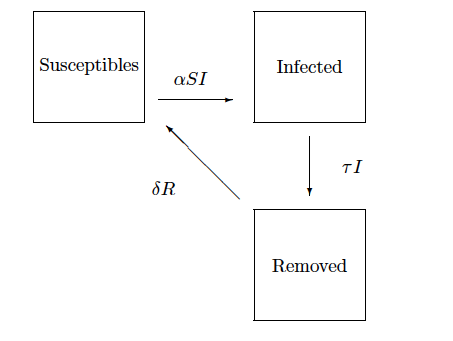Here α is the rate at which susceptible people become infected by contact with an infected person. The constants τ and δ represent the rates at which infected (resp. removed) individuals become removed (resp. susceptible). We will assume α > 0, τ > 0 and that δ ≥ 0. It is important to consider the case in which δ > 0 in view of the possibility that people who have recovered from an infection lose their immunity over time.

We can normalize our time units to make τ = 1. One-time unit then equals the average time it takes an infected person to become removed. The differential equations are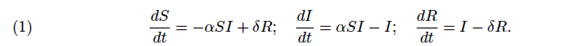It follows that S(t) + I(t) + R(t) does not vary with time, so we can normalize this sum to be 1. Eliminating R(t) leads to the two variable system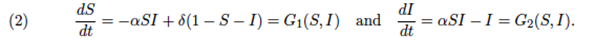in which S(t) and I(t) are the proportions of the population at time t which are susceptible and infected, respectively.

The equilibria of the system occur where G1 and G2 vanish. An equilibrium is stable if all initial positions sufficiently close to the equilibrium lead to trajectories that tend back to the equilibrium. An equilibrium is linearly stable if it becomes stable when we replace G1 and G2 by their first order Taylor approximations near the equilibrium. This is equivalent to requiring that the eigenvalues of the Jacobian matrixhave negative real parts when this matrix is evaluated at the equilibrium.

##### 1. The case δ = 0.

The case δ=0 is the most classical one (c.f. [3, Lecture 1]). The equilibria are precisely the points (S(0),0) with 0 ≤ S(0) ≤ 1, and none are stable or linearly stable. The function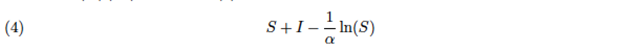is constant on trajectories. These are illustrated by the following vector fields when α = 0.5, α = 1 and α = 2.5: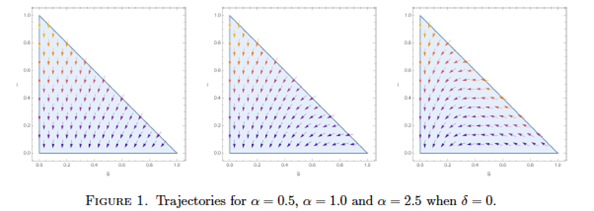One sees from (2) that dS/dt ≤ 0, so S(t) is non-increasing. Suppose I(0) > 0. As shown in [3, §1.2], when S(0) < 1/α, I(t) is monotonically decreasing with limit 0. If S(0) > 1/α, I(t) initially increases, and the constant 1/α is the value of S at which I achieves its maximum along a trajectory and then decreases with limit 0. For this reason, 1/α is often referred to as the herd immunity point. It is the maximal fraction of the population that can be susceptible if an infection is to decrease from the start. Since limt I(t) = 0, the fact that (4) is constant makes it possible to determine limt S(t) for any initial position (S(0),I(0)).

While (S(0),0) is never a stable equilibrium, one can use that (4) is constant to show that it is approximately stable when S(0) < 1/α in the following sense. Suppose 0 ≤ S(0) < 1/α, and that one starts a new trajectory at some distance ε from the equilibrium (S(0),0). If ε is sufficiently small, the limit of the resulting trajectory will be a new equilibrium whose distance from the original equilibrium is bounded by cε/|1-αS(0)| for a constant c independent of S(0).

Suppose now that instead, S(0) > 1/ α. Then the trajectory that starts at a non-equilibrium point within ε of (S(0),0) will have a limit with an S-value less than 1/α. So the new equilibrium will always have distance at least S(0)-1/α from the initial one. Thus there is no constant c as above for all sufficiently small ε.

##### 2. The case δ > 0.

We now consider the case δ > 0, so that removed individuals can become susceptible again. There is an equilibrium at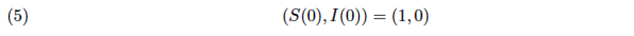which is linearly stable if and only if α < 1 and stable if and only if α ≤ 1. If α > 1, there is another equilibrium at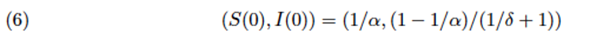which is linearly stable.

The trajectories for δ = 0.6 are illustrated by the following vector fields when α = 0.5, α = 1 and α = 2.5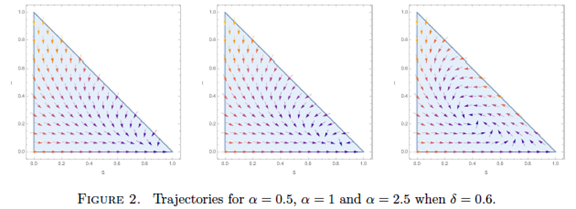Notice that when α ≤ 1, the trajectories all have limit (1,0). For each α > 1, the equilibrium at (6) will be a stable spiral, a degenerate node, or a stable node. All three possibilities in fact arise for each α>1 from different values of δ > 0. These cases correspond to the eigenvalues of the Jacobian matrix at the equilibrium having negative real part and being, respectively, non-real, equal and real, or unequal and real. In all cases, the existence of attractive equilibria implies that all continuous functions of S and I that are constant on trajectories are in fact constant.

While these models are attractive mathematically, one should be cautious when applying them. They do not take into account births and deaths, for example, or the fact that in the process of becoming removed, some individuals die and some recover. The greatest uncertainty arises from the variability of parameters such as the transmissibility coefficient as a result of behavioral changes. One natural approach is to treat parameters as probability distributions. This leads to the theory of stochastic differential equations. See [1, §5.2.2] for an example pertaining to the theory of epidemics.

### Prof. Ted Chinburg

Department of Mathematics,